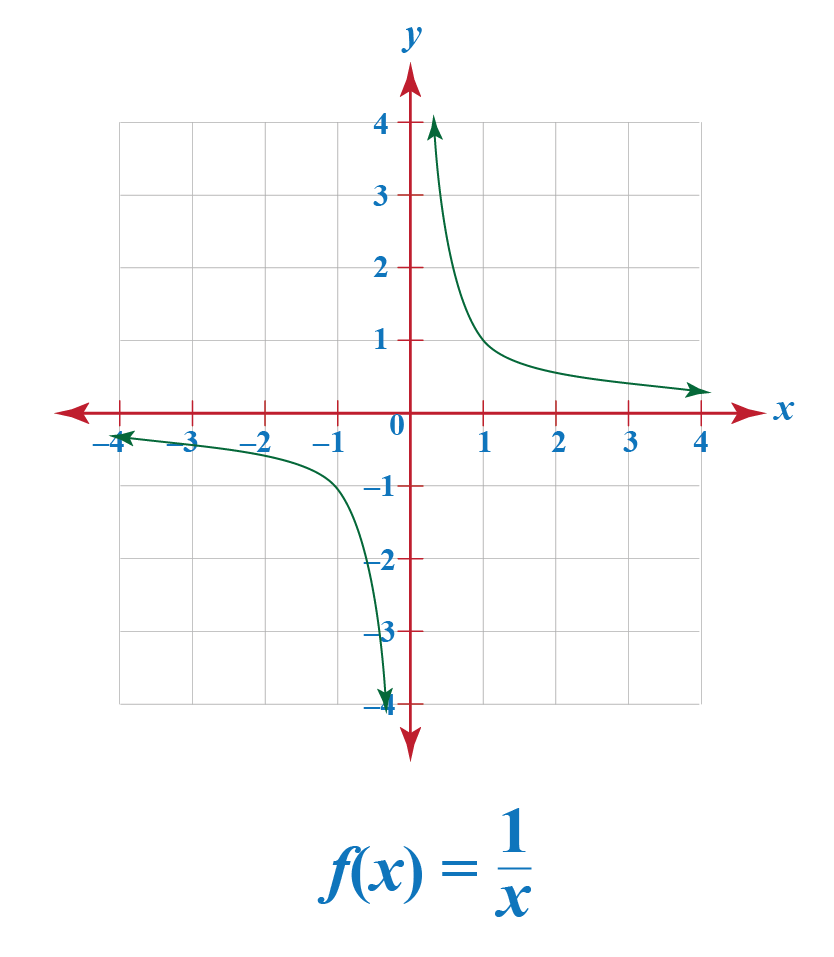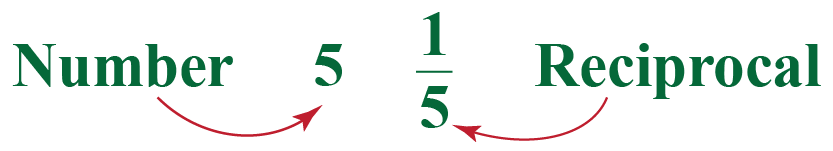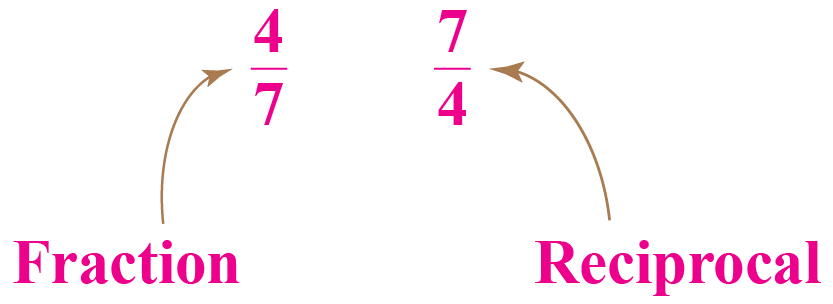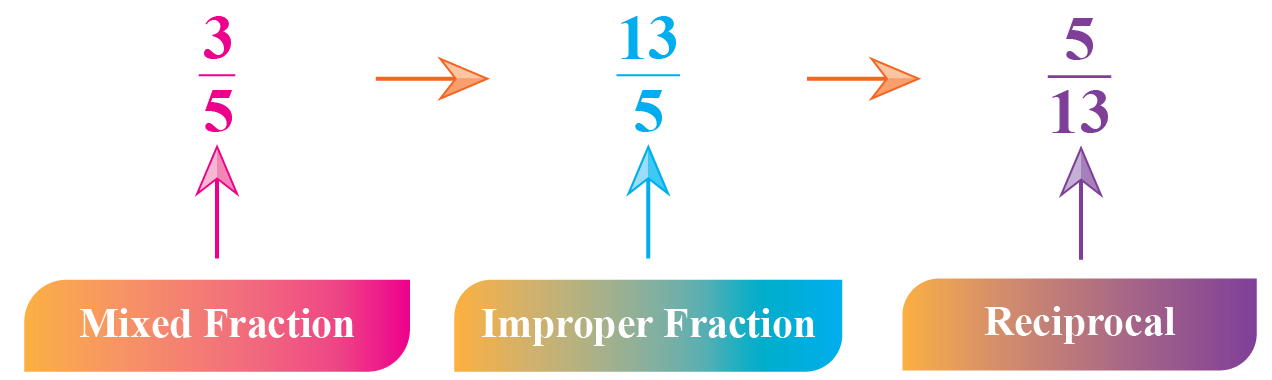# Reciprocal Function

Reciprocal Function

A simple definition of reciprocal is 1 divided by a given number. When we multiply the reciprocal of a number with the number, the result is always 1. Due to this reason, it is also called the multiplicative inverse.The mini-lesson discusses the reciprocal function definition, its domain and range, graphing of the reciprocal function, solved examples on reciprocal functions, and interactive questions.

Try out the reciprocal function calculator given below.

## Lesson Plan

 1 What Is Reciprocal Function? 2 Important Notes on Reciprocal Functions 3 Thinking out of the Box! 4 Solved Examples on Reciprocal Functions 5 Interactive Questions on Reciprocal Functions

## What Do You Mean by Reciprocal Functions?

For a given function \begin{align} f(x)\end{align}, the reciprocal is defined as \begin{align} \dfrac{a}{x-h} + k \end{align}, where the vertical asymptote is \begin{align} x=h \end{align} and horizontal asymptote is \begin{align} y = k \end{align}. The reciprocal function is also called the "Multiplicative inverse of the function".

The common form of a reciprocal function is \begin{align}y = \dfrac{k}{x} \end{align}, where \begin{align}k \end{align} is any real number and \begin{align}x \end{align} can be a variable, number or a polynomial.

The reciprocal of a number is a number which when multiplied with the actual number produces a result of 1

For example, let us take the number \begin{align} 2 \end{align}. The reciprocal is,

When \begin{align} \dfrac{1}{2}\end{align}

Also, when we multiply the reciprocal with the original number we get 1

\begin{align} \dfrac{1}{2} \times 2 = 1\end{align}

Some examples of reciprocal functions are,

\begin{align}f(x) &= \dfrac{1}{5} \\ f(x) &= \dfrac{2}{x^2} \\ f(x) &= \dfrac{3}{x-5}\end{align}

In the exponent form, the reciprocal function is written as,

 \begin{align}f(x) = a(x-h)^{-1} + k \end{align}

## Characteristics of Reciprocal Functions

• Reciprocal functions are in the form of a fraction. The numerator is a real number and the denominator is either a number or a variable or a polynomial.
• The reciprocal \begin{align} x \end{align} is \begin{align} \dfrac{1}{x}\end{align}
• The denominator of a reciprocal function cannot be 0. For example, \begin{align} f(x) = \dfrac{3}{x-5}\end{align} cannot be 0, which means 'x' cannot take the value 5.
• The domain and range of the reciprocal function \begin{align} f(x) = \dfrac{1}{x}\end{align} is the set of all real numbers except 0.
• The graph of the equation \begin{align} f(x) = \dfrac{1}{x}\end{align} is symmetric with the equation \begin{align} y = x \end{align}.

## How to Graph Reciprocal Functions?

There are many forms of the reciprocal functions. One of them is of the form \begin{align} \dfrac{k}{x}\end{align}. Here 'k' is  real number and the value of 'x' cannot be 0. Now let us draw the graph for the function \begin{align}f(x) = \dfrac{1}{x} \end{align} by taking different values of x and y.

 x -3 -2 -1 -1/2 -1/3 1/3 1/2 1 2 3 y -1/3 -1/2 -1 -2 -3 3 2 1 1/2 1/3For a reciprocal function \begin{align} f(x) = \dfrac{1}{x} \end{align}, 'x' can never be 0 and so  \begin{align} \dfrac{1}{x} \end{align} can also not be equal to 0. Therefore the domain and range of reciprocal function are as follows.

 Domain is the set of all real numbers except 0,since \begin{align}\dfrac{1}{0} \end{align} is undefined \begin{align}{\{x \in R\: | \:x \neq 0\}} \end{align} Range is also the set of all real numbers. \begin{align}{\{x \in R\: | \:x \neq 0\}} \end{align}

From the graph we observe that they never touch the x-axis and y-axis.

The y-axis is said to be the vertical asymptote as the curve gets very closer but never touches it.

Also, the x-axis is the horizontal asymptote as the curve never touches the x-axis.Think Tank
1. Sketch the graph of the reciprocal function f(x) = -1/x and find how it is related to the function f(x) = 1/x.

## How Do You Solve  Reciprocal Functions?

### Reciprocal of a Number

To find the reciprocal we divide the number, variable, or expression by 1For example,

Reciprocal of 6 is \begin{align} &\dfrac{1}{6}\end{align}

### Reciprocal of a Variable

The reciprocal of a variable 'y' can be found by dividing the variable by 1

For example,

Reciprocal of y is \begin{align} &\dfrac{1}{y}\end{align}

### Reciprocal of a Expression

The reciprocal of an expression can be found by exchanging the positions of numerator and denominator.

Examples are,

Reciprocal of  \begin{align}\dfrac{x}{x-4}\end{align} is \begin{align}\dfrac{x-4}{x}\end{align}

### Reciprocal of a Fraction

Reciprocal of a fraction can be obtained by flipping the places of numerator and denominator.For example,

Reciprocal of \begin{align}\dfrac{5}{8}\end{align} is \begin{align}\dfrac{8}{5}\end{align}.

### Reciprocal of a Mixed Fraction

Reciprocal of a mixed fraction can be obtained by finding the improper fraction and then finding its reciprocal.

For example,

To find the reciprocal of \begin{align}3\dfrac{3}{4}\end{align}, we find the improper fraction which is \begin{align}\dfrac{15}{4}\end{align} and now find the reciprocal \begin{align}\dfrac{4}{15}\end{align}Functions
grade 10 | Questions Set 2
Functions
Functions
grade 10 | Questions Set 1
Functions
More Important Topics
Numbers
Algebra
Geometry
Measurement
Money
Data
Trigonometry
Calculus
More Important Topics
Numbers
Algebra
Geometry
Measurement
Money
Data
Trigonometry
Calculus
Learn from the best math teachers and top your exams

• Live one on one classroom and doubt clearing
• Practice worksheets in and after class for conceptual clarity
• Personalized curriculum to keep up with school